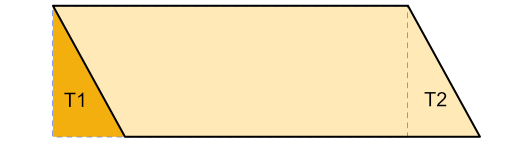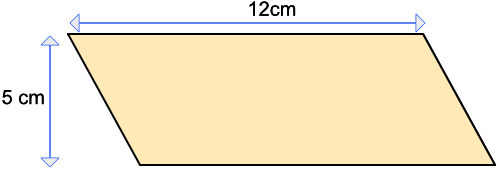Area of a Parallelogram

## Area of a Parallelogram

The area of a parallelogram is base x vertical height.

This can be deduced from the area of a rectangle, which is A = bhMove a triangle from one end of a parallelogram (from T2), and position at the other end (T1). The area of the parallelogram is the same as the area of the rectangle.

Note that it is the vertical distance that provides the height.

## Example 1

What is the area of the parallelogram?Area of a parallelogram is base x vertical height

A = 12 x 5

A = 60